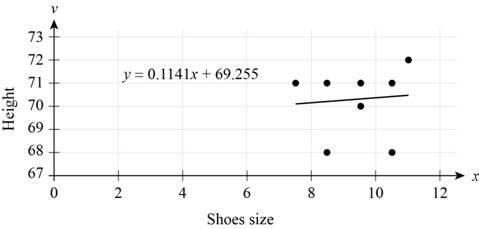# The shoes size and height of people in classroom by surveying and correlation.### Precalculus: Mathematics for Calcu...

6th Edition
Stewart + 5 others
Publisher: Cengage Learning
ISBN: 9780840068071### Precalculus: Mathematics for Calcu...

6th Edition
Stewart + 5 others
Publisher: Cengage Learning
ISBN: 9780840068071

#### Solutions

Chapter 1, Problem 12P

(a)

To determine

## The shoes size and height of people in classroom by surveying and correlation.

Expert Solution

The shoes size and height of people height is linear.

### Explanation of Solution

The data shoes size and height of people by surveying in the class room is,

 Shoe size Height 9.5 71 9.5 70 10.5 68 10.5 71 11 72 7.5 71 8.5 68 8.5 71 9.5 71

Table (1)

The scatter plot of above data is shown below from Table (1).Figure (1)

From Figure (1), the life height and shoes size are shown on coordinate and abscissa. The plot is represented by Cartesian coordinate with the independent variable x on the horizontal axis and dependent variable y on the vertical axis. The Figure (1) gives the linear equation and linear line. So, the above analysis shows that there is a linear correlation between shoes size and height.

Thus, the shoes size and height of people is linear.

(b)

To determine

### The correlation coefficient.

Expert Solution

The correlation coefficient is 7.12 .

### Explanation of Solution

The data shoes size and height of people by surveying in the class room is,

 Shoe size Height 9.5 71 9.5 70 10.5 68 10.5 71 11 72 7.5 71 8.5 68 8.5 71 9.5 71

To evaluate the above data in the form of x and y .

 x y xy x2 y2 9.5 71 674.5 90.25 5041 9.5 70 66.5 90.25 4900 10.5 68 714 110.25 4624 10.5 71 745.5 110.25 5041 11 72 792 121 5184 7.5 71 532.5 56.25 5041 8.5 68 578 72.25 4624 8.5 71 603.5 72.25 5041 9.5 71 764.5 90.25 5041 ∑x=85 ∑y=633 ∑xy=5471 ∑x2=813 ∑y2=44537

Where,

• x is the size of the shoes.
• y is the height of people.

Formula to calculate correlation coefficient is,

C=xy(x)(y)n(x2)(x)2n(x2)(x)2

Where,

• C is the correlation coefficient.
• n is the number of persons.

Substitute 5471 for xy , 633 for y , 813 for x2 , 44537 for y2 and 85 for x to find the C .

C=9×547185×6339(813)(85)29(44537)(633)2=820.5115.09=7.12

Thus, the correlation coefficient is 7.12 .

### Have a homework question?

Subscribe to bartleby learn! Ask subject matter experts 30 homework questions each month. Plus, you’ll have access to millions of step-by-step textbook answers!